# Trees and Cotrees of an Electric Network (Graph Theory)

A tree of electric network is set of branches which is a set of branches which contains all the nodes of the network but does not form any closed path. It is similar to what a network topology is to a communications network.

Let us explain the tree of electric network as defined above.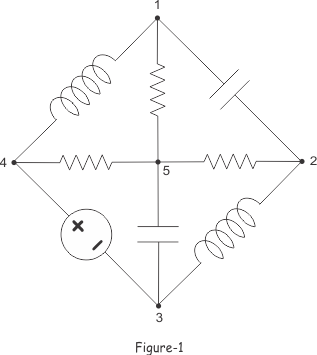The above figure-1, shows an electric network with five nodes 1,2,3,4 and 5.

Now, if we remove the branches 1-2, 2-3, 3-4 and 4-1 from the circuit, we will get, the graph as shown below in figure-2.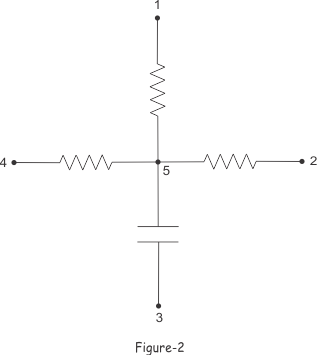The above graph as shown in the figure-2, contains all the five nodes of the network, but does not from any closed path. This is an example of tree of electric network.

In this way numbers of such tree can be formed in a single electric circuit, which contains same five nodes without containing any closed loop.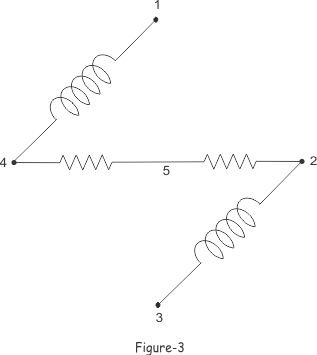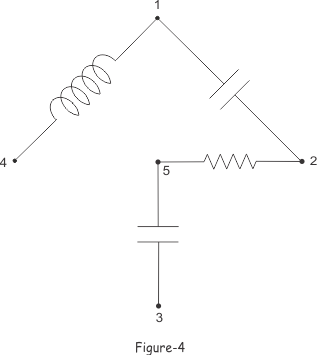The branches of a tree are also known as twigs.
In figure-2, figure-3 and figure-4 we can see that, there are four twigs or branches of tree in each tree of that electric network. The number of nodes in the network is 5.
So, in this case,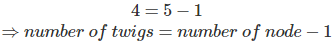This is a general equation for all trees of any electric network. The general equation is normally written as,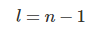Where, l is the number of branches in a tree and n is the number of nodes in the network from which the trees are formed.

## Cotrees of Electric Network

When, a graph is formed from an electric network, some selective branches are taken. The branches of the network which are not in tree formation are referred as links or chords. The graph formed by these links or chords is called cotree. Cotree can be closed or opened depending upon the links.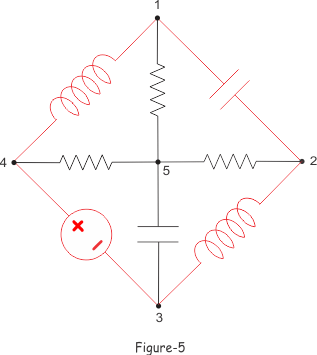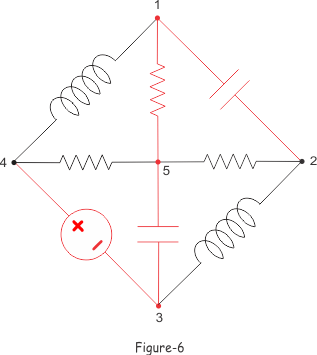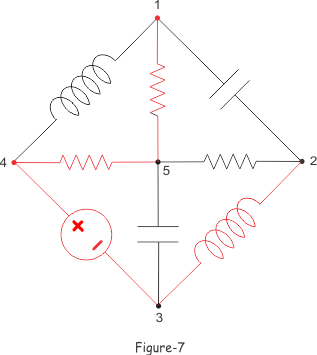The cotrees are shown in the above figures by red colour. It is found from figure-5, figure-6 and figure-7 that, the sum of number of branches of tree and its cotree is the total number branches of electric network.
So, if number of links of a cotree is l’, then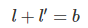Where, l is the number of twigs in the tree and b is the number of branches in a network. So,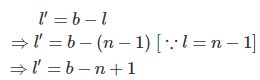Where, n is the number of nodes in the electric network.

## Properties of Tree of Electric Netwrok

• A tree consists of all the nodes of the electric network.
• A tree has the number of branches which is less than 1 of number of nodes of the electric network.
• A tree must not have any closed path in any part of it.
• There may be many different possible trees in same electric network.
• The sum of the number of branches in a tree and number of branches of its cotree is equal to the total number of branches of their electric network.
• The number of independent Kirchhoff Voltage Law equations can be formed for an electric network is equal to its number of links or chords of cotree.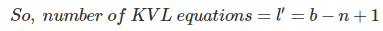• The number of independent Kirchhoff Current Law equations can be formed for an electric network is equal to the its number of twigs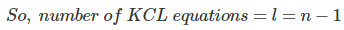Want To Learn Faster? 🎓
Get electrical articles delivered to your inbox every week.
No credit card required—it’s 100% free.